# Formula Class

#### Yogesh.ExcelXml

A Sandcastle Documented Class Library Formula Class
NamespacesYogesh.ExcelXmlFormula
Formula is a formula builder class which can be stored directly in a cell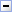Declaration Syntax
 C# Visual Basic Visual C++
`public class Formula`
`Public Class Formula`
`public ref class Formula`Members
 All Members Constructors Methods Properties Public Protected Instance Static Declared Inherited
Icon Member Description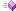Formula(String)
Constructs a formula without any parametersFormula(String, Range)
Constructs a formula and adds a range as the first parameterFormula(String, String)
Constructs a formula and adds a string as the first parameterFormula(String, Formula)
Constructs a formula and adds another formula as the first parameterFormula(String, Range, Predicate<(Of <(Cell>)>))
Constructs a formula and adds a filtered range as the first parameterAdd(Range)
Adds a range as a parameter in a formulaAdd(String)
Adds a string as a parameter in a formulaAdd(Formula)
Adds another formula as a parameter in a formulaAdd(Range, Predicate<(Of <(Cell>)>))
Adds a filtered range as a parameterEquals(Object)
Determines whether the specified Object is equal to the current Object.
(Inherited from Object.)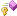Finalize()()()
Allows an Object to attempt to free resources and perform other cleanup operations before the Object is reclaimed by garbage collection.
(Inherited from Object.)GetHashCode()()()
Serves as a hash function for a particular type. GetHashCode()()() is suitable for use in hashing algorithms and data structures like a hash table.
(Inherited from Object.)GetType()()()
Gets the Type of the current instance.
(Inherited from Object.)MemberwiseClone()()()
Creates a shallow copy of the current Object.
(Inherited from Object.)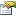MustHaveParameters
Check to force parameters in functionParameters
Readonly list of formula paramtersToString()()()
Returns a String that represents the current Object.
(Inherited from Object.)Inheritance Hierarchy
 Object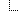Formula

Assembly: Yogesh.ExcelXml (Module: Yogesh.ExcelXml) Version: 2.89.501.2158